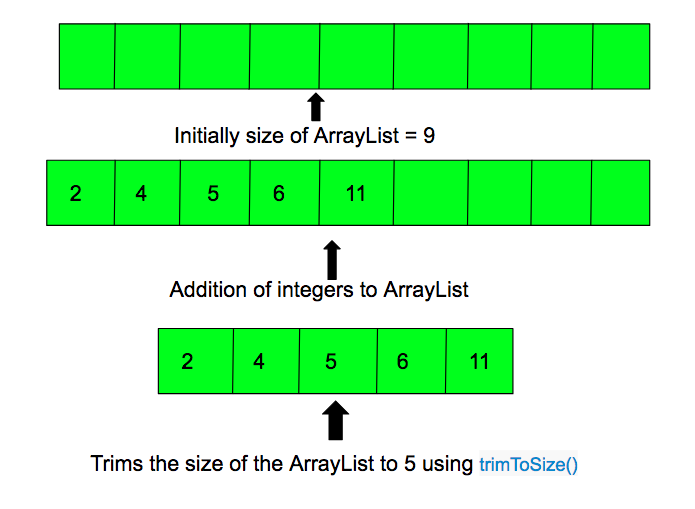Open In App

# ArrayList trimToSize() in Java with example

The trimToSize() method of ArrayList in Java trims the capacity of an ArrayList instance to be the list’s current size. This method is used to trim an ArrayList instance to the number of elements it contains.

Syntax:

`trimToSize()`

Parameter: It does not accepts any parameter.

Return Value: It does not returns any value. It trims the capacity of this ArrayList instance to the number of the element it contains.

Errors and Exceptions: It does not have any errors and exceptions.Below program illustrate the trimTosize() method:

 `// Java code to demonstrate the working of``// trimTosize() method in ArrayList`` ` `// for ArrayList functions``import` `java.util.ArrayList;`` ` `public` `class` `GFG {``    ``public` `static` `void` `main(String[] args)``    ``{`` ` `        ``// creating an Empty Integer ArrayList``        ``ArrayList arr = ``new` `ArrayList(``9``);`` ` `        ``// using add(), add 5 values``        ``arr.add(``2``);``        ``arr.add(``4``);``        ``arr.add(``5``);``        ``arr.add(``6``);``        ``arr.add(``11``);`` ` `        ``// trims the size to the number of elements``        ``arr.trimToSize();`` ` `        ``System.out.println(``"The List elements are:"``);`` ` `        ``// prints all the elements``        ``for` `(Integer number : arr) {``            ``System.out.println(``"Number = "` `+ number);``        ``}``    ``}``}`

Output:

```The List elements are:
Number = 2
Number = 4
Number = 5
Number = 6
Number = 11
```